# Randomized Circle Packing

Date started: October 2019
Leads: Roger Antonsen

###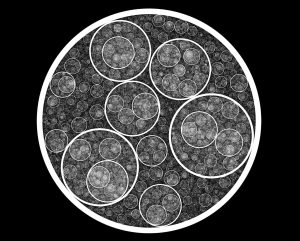Abstract

Here are some experiments (work-in-progress) with randomized circle packings. The algorithm is deceptively simple: Start with an initial circle and repeatedly choose a point, according to some algorithm, inside of the initial circle and add the largest circle possible having this point as its center. By constraining the way the points are chosen, a variety of different figures emerges. How many circles are enclosing the point? What is the angle of the point? How far from the center is the point? If points are only allowed to be added given that certain properties are satisfied, we obtain different visual effects.

### Media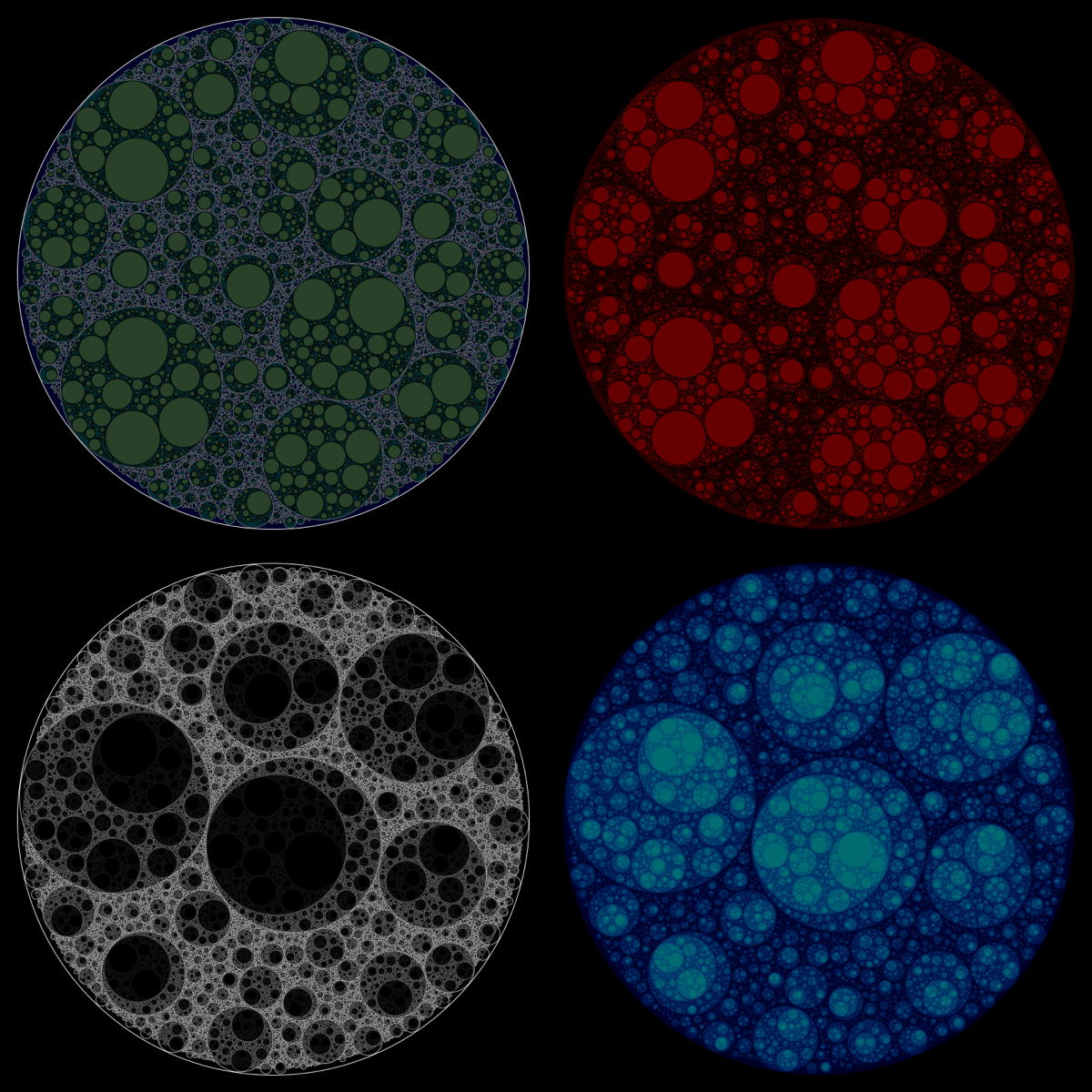These emerge from picking points at random and constraining the depth – the number of enclosing circles – to certain numbers.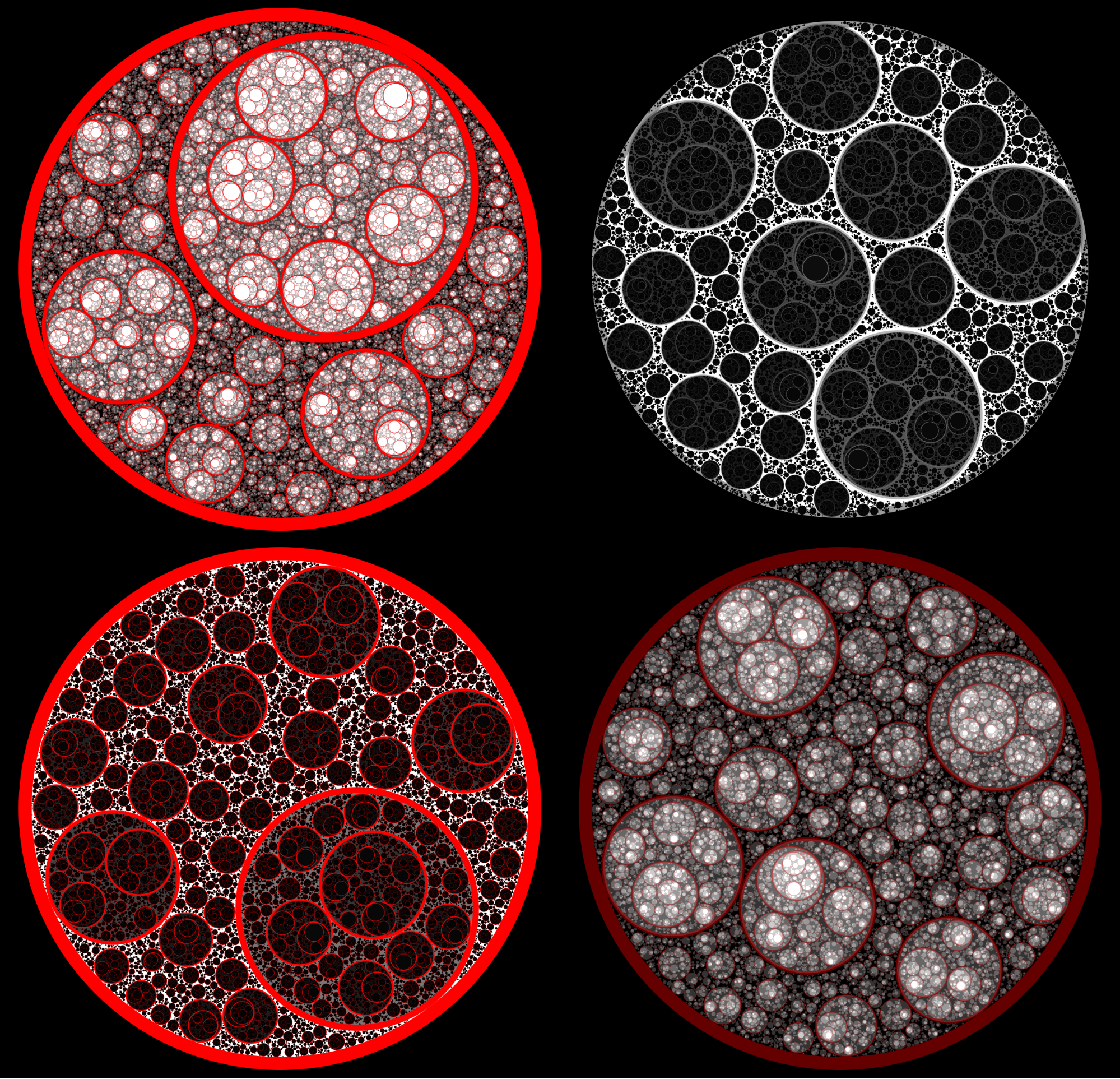By allowing a circle to have both an inner and an outer radius, a certain visual effect is achieved.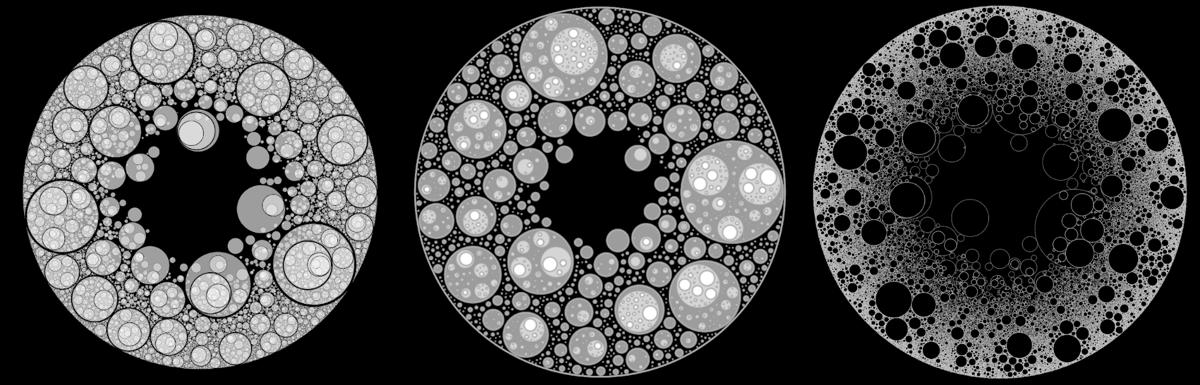These are obtained by picking points closer to the circumference more often than points close to the center.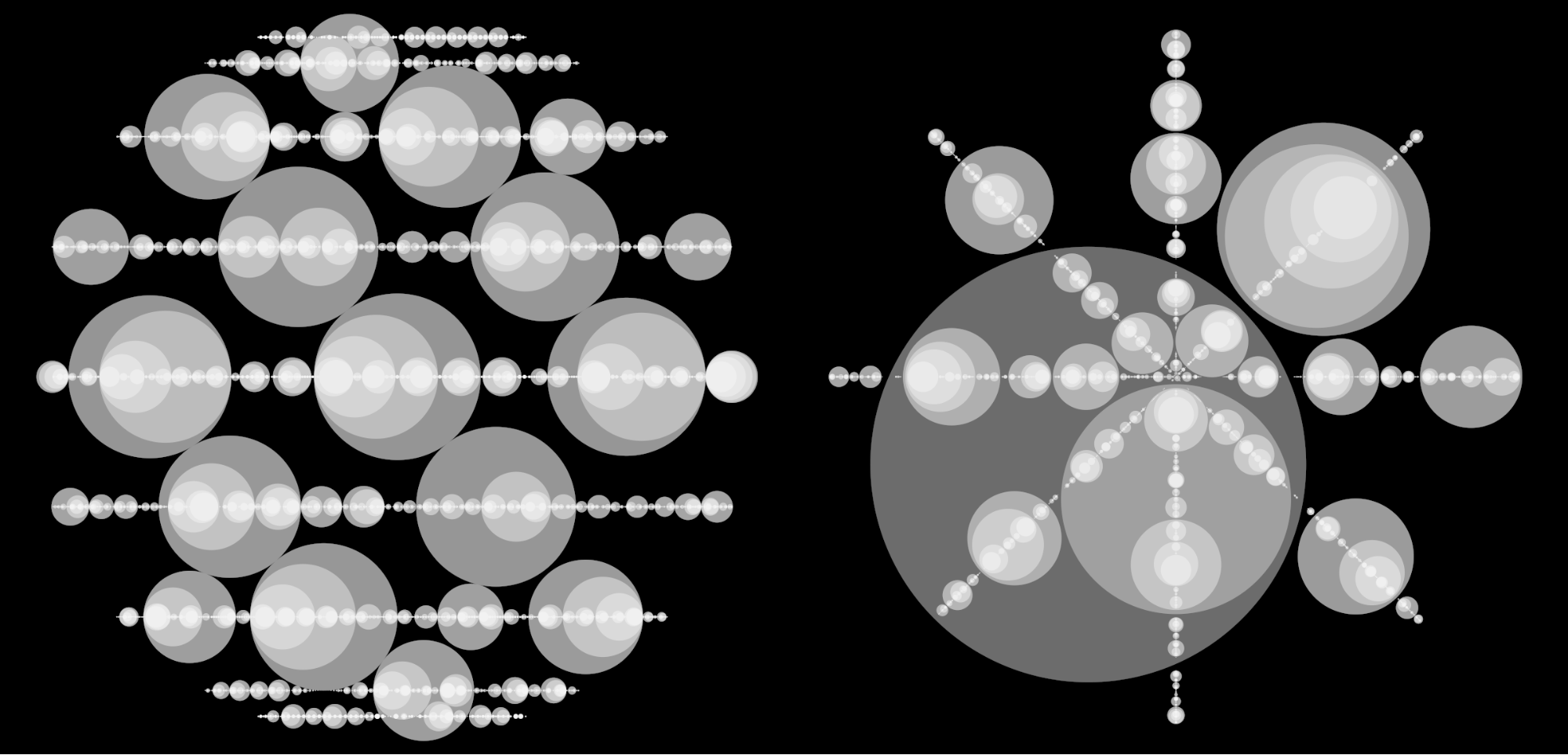These are obtained by picking points on given horizontal lines (left) or given radial lines (right).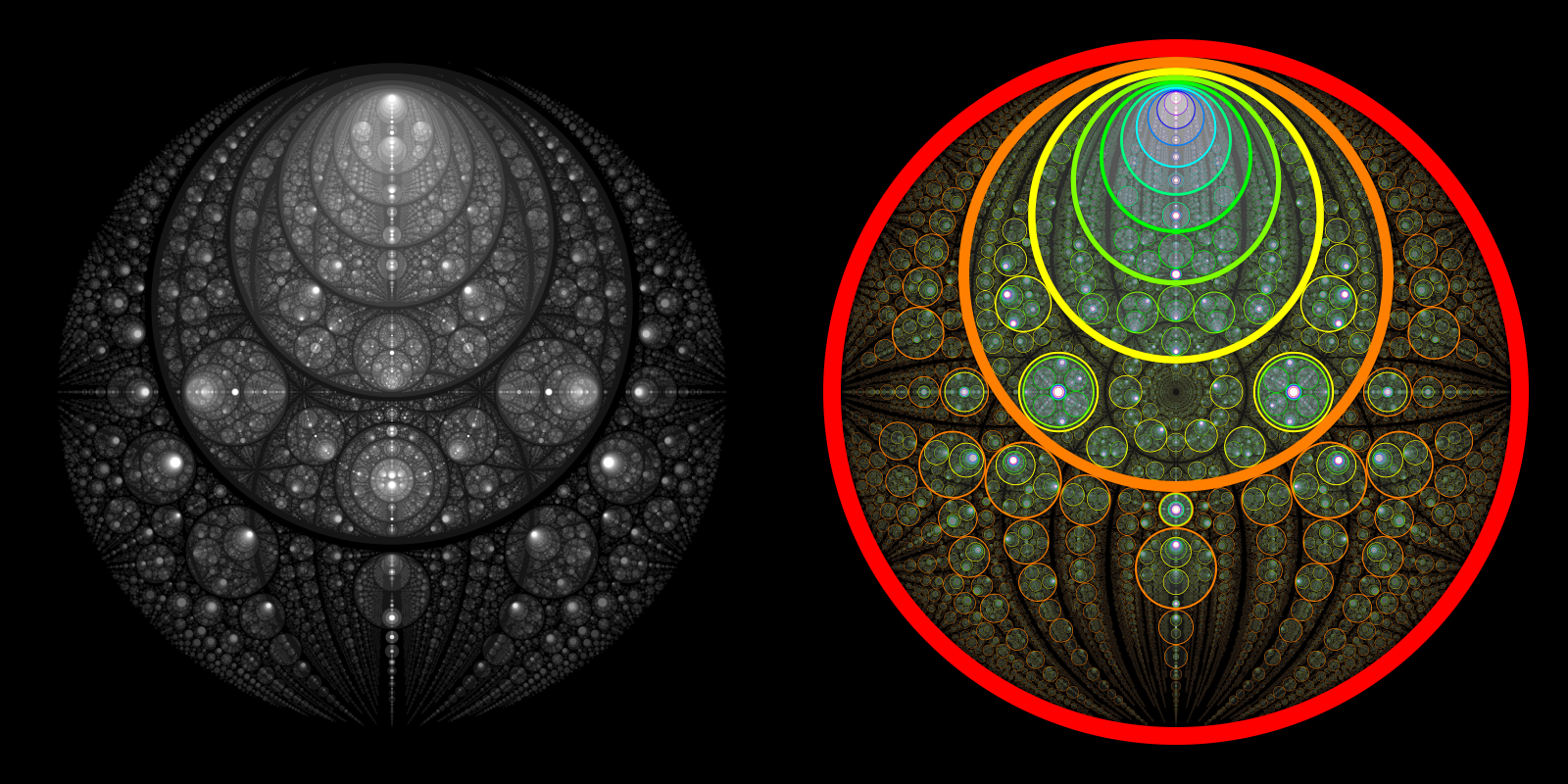These – and the rest of the figures – are obtained by varying the ways with which the radii and the angles as chosen for each additional point. These have 2-fold symmetry.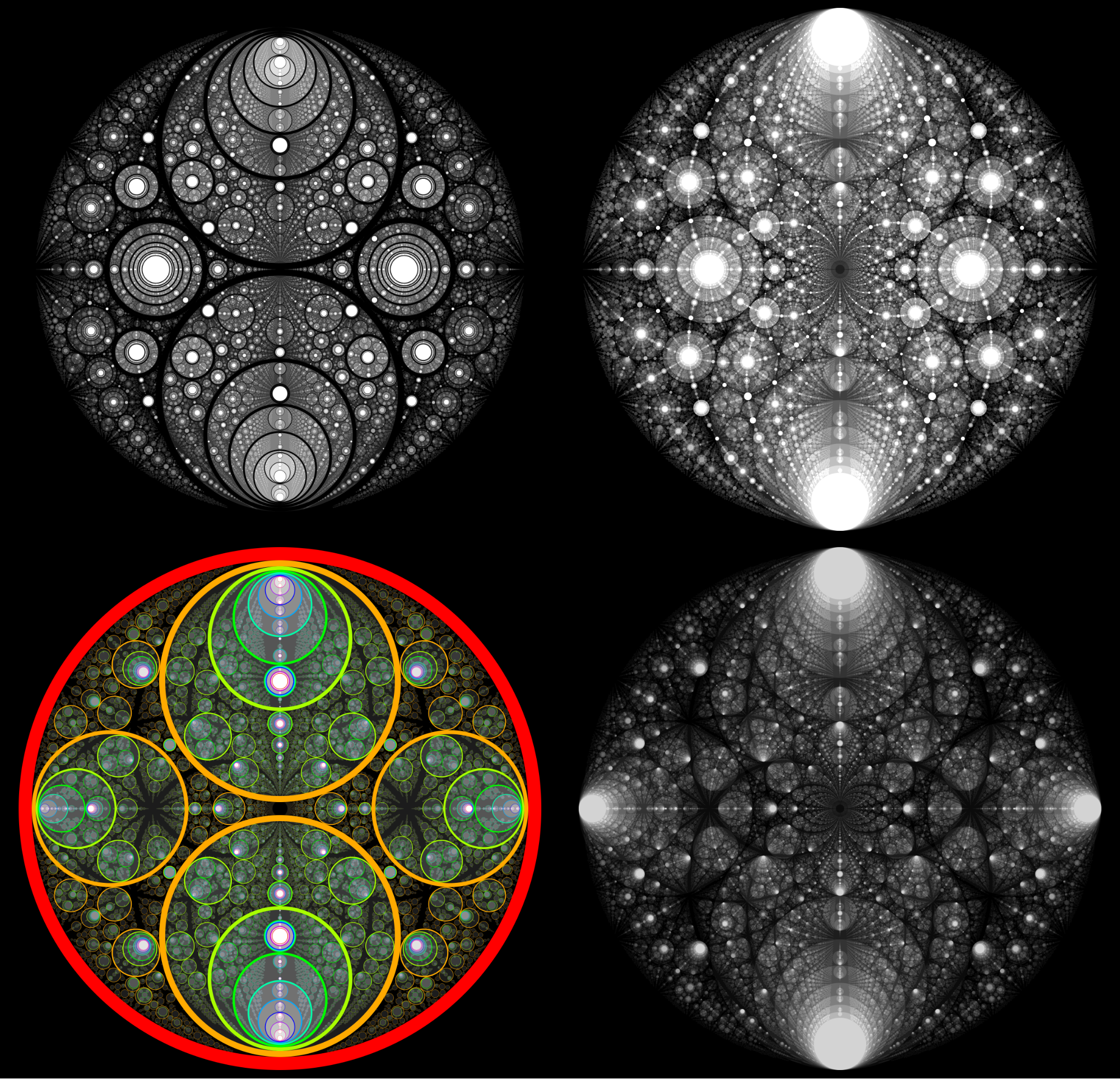Here are some more with 2-fold symmetry.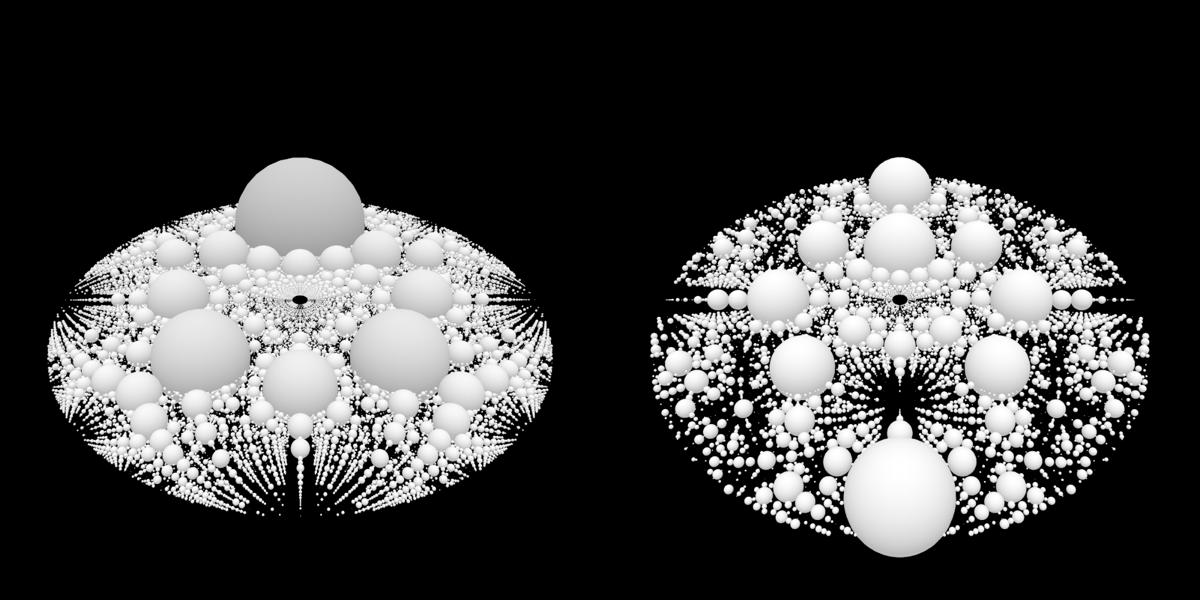These are simple 3D extensions of some of the above figures.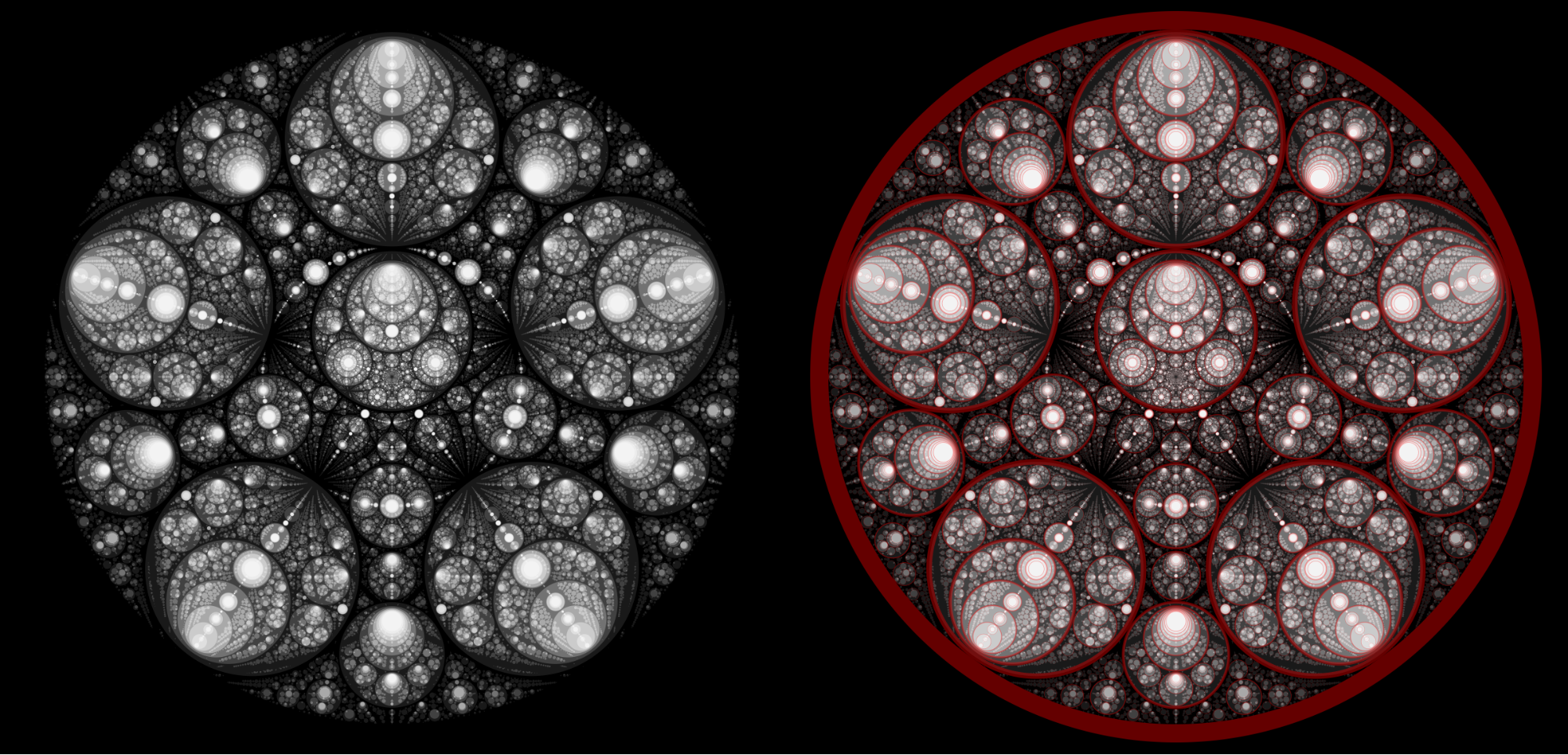Here are some with almost 5-fold symmetry.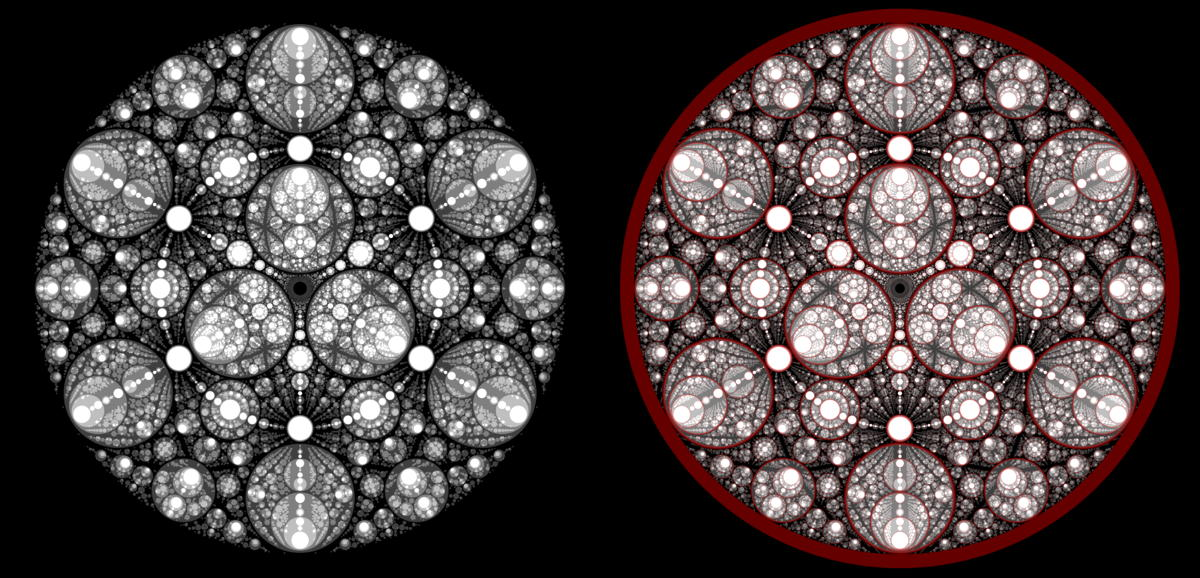Here are some with almost 6-fold symmetry.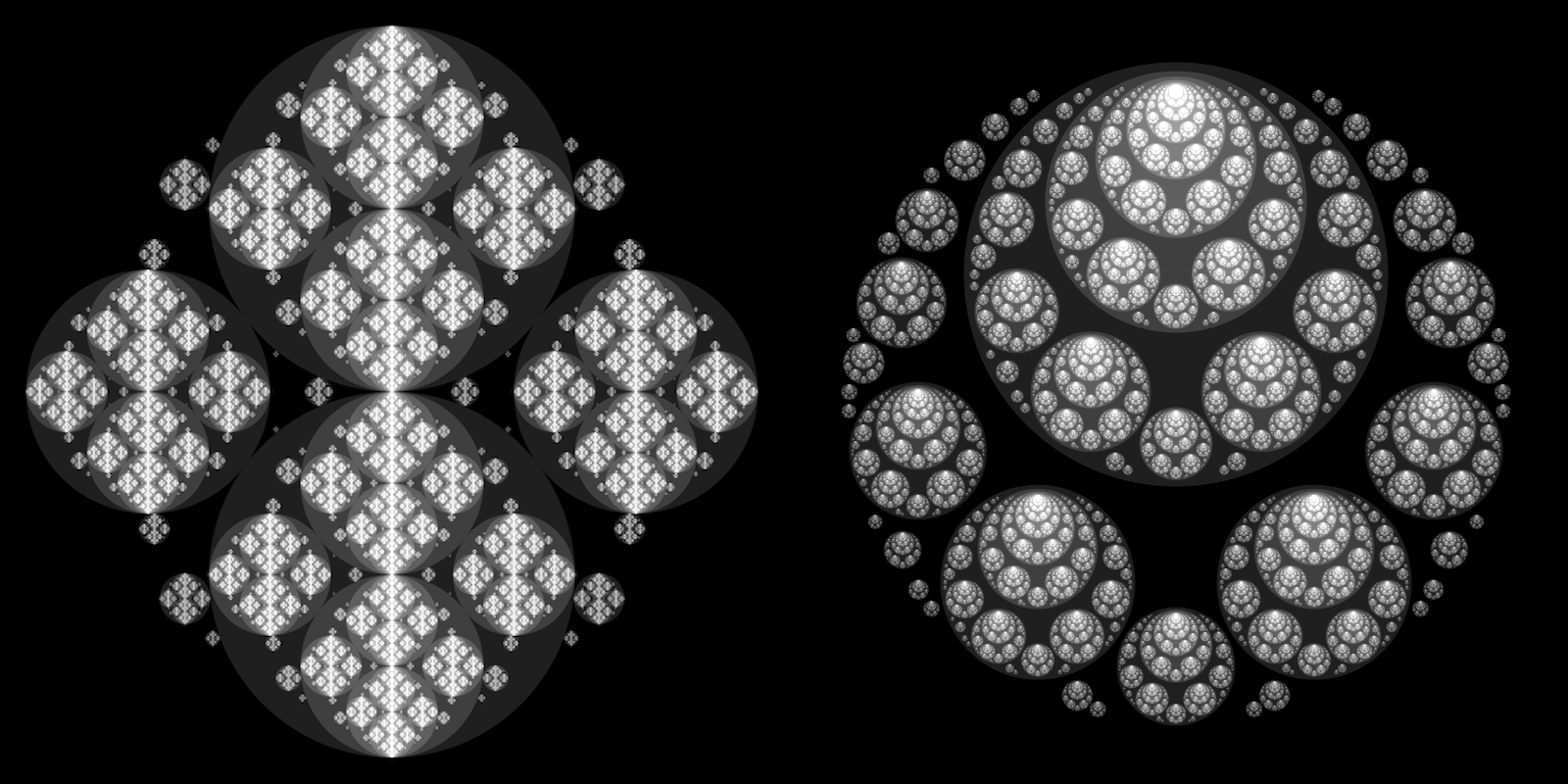These are some experiments with recursive addition of points.